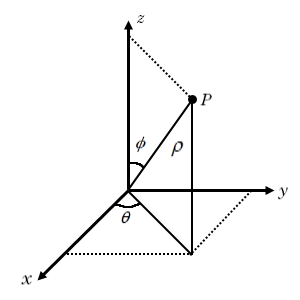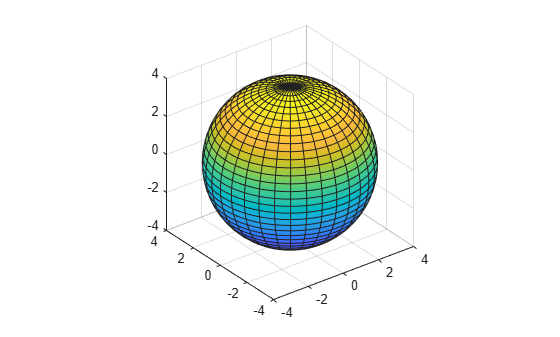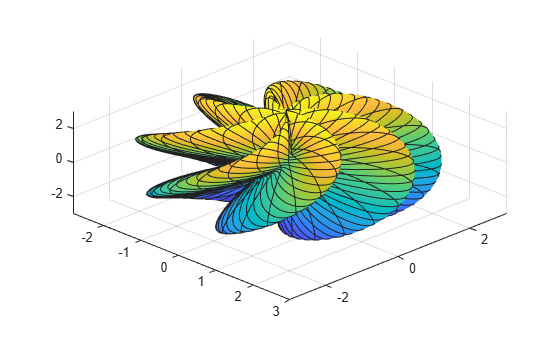# Transform Spherical Coordinates to Cartesian Coordinates and Plot Analytically

This example shows how to transform a symbolic expression from spherical coordinates to Cartesian coordinates, and plot the converted expression analytically without explicitly generating numerical data.

In the spherical coordinate system, the location of a point $P$ can be characterized by three variables. Different textbooks have different conventions for the variables used to describe spherical coordinates. For these examples, this convention is used:

• The radial distance $\rho$

• The azimuthal angle $\theta$

• The polar angle $\varphi$The transformation of the point $P$ from spherical coordinates $\left(\rho ,\theta ,\varphi \right)$ to Cartesian coordinates $\left(x,y,z\right)$ is given by

`$\begin{array}{l}\mathit{x}=\rho \text{\hspace{0.17em}}\mathrm{sin}\text{\hspace{0.17em}}\varphi \text{\hspace{0.17em}}\mathrm{cos}\text{\hspace{0.17em}}\theta ,\\ \mathit{y}=\rho \text{\hspace{0.17em}}\mathrm{sin}\text{\hspace{0.17em}}\varphi \text{\hspace{0.17em}}\mathrm{sin}\text{\hspace{0.17em}}\theta ,\\ \mathit{z}=\rho \text{\hspace{0.17em}}\mathrm{cos}\text{\hspace{0.17em}}\varphi .\end{array}$`

By transforming symbolic expressions from spherical coordinates to Cartesian coordinates, you can then plot the expressions using Symbolic Math Toolbox™ graphics functions, such as `fplot3` and `fsurf`.

### Plot a Point and Its Projections

Plot the point $P$ that is located at $\left(\rho ,\theta ,\varphi \right)=\left(1,1.2,0.75\right)$.

Transform the spherical coordinates to Cartesian coordinates $\left({x}_{P},{y}_{P},{z}_{P}\right)$. Because the converted coordinates contain numerical values, use `plot3` to plot the point.

```rho = 1; theta = 1.2; phi = 0.75; x_P = rho*sin(phi)*cos(theta); y_P = rho*sin(phi)*sin(theta); z_P = rho*cos(phi); plot3(x_P,y_P,z_P,'ko','MarkerSize',10,'MarkerFaceColor','k') hold on```

Label each axis in the plot, change the line of sight, and set the axis scaling to use equal data units.

```xlabel('x') ylabel('y') zlabel('z') view([75 40]) axis equal;```

Next, plot the line projection of the point $P$ to the origin. This line projection in spherical coordinates is parameterized by $\left(r,1.2,0.75\right)$, with $r$ ranging from $0$ to $1$. Specify this line parameterization as symbolic expressions, and plot it by using `fplot3`.

```syms r xr = r*sin(phi)*cos(theta); yr = r*sin(phi)*sin(theta); zr = r*cos(phi); fplot3(xr,yr,zr,[0 rho],'k')```

Plot the vertical line projection to the $xy$-plane. This line projection in Cartesian coordinates is parameterized by $\left({x}_{P},{y}_{P},z\right)$, with $z$ ranging from $0$ to ${z}_{P}$.

```syms z fplot3(sym(x_P),sym(y_P),z,[0 z_P],'k')```

Plot the top horizontal line projection to the $z$-axis. This line projection in Cartesian coordinates is parameterized by $\left(r\phantom{\rule{0.2222222222222222em}{0ex}}\mathrm{sin}\varphi \phantom{\rule{0.2222222222222222em}{0ex}}\mathrm{cos}\theta ,r\phantom{\rule{0.2222222222222222em}{0ex}}\mathrm{sin}\varphi \phantom{\rule{0.2222222222222222em}{0ex}}\mathrm{sin}\theta ,{z}_{P}\right)$, with $r$ ranging from $0$ to $1$.

`fplot3(xr,yr,sym(z_P),[0 rho],'k--')`

Plot the bottom horizontal line projection to the $z$-axis. This line projection in Cartesian coordinates is parameterized by $\left(r\phantom{\rule{0.2222222222222222em}{0ex}}\mathrm{sin}\varphi \phantom{\rule{0.2222222222222222em}{0ex}}\mathrm{cos}\theta ,r\phantom{\rule{0.2222222222222222em}{0ex}}\mathrm{sin}\varphi \phantom{\rule{0.2222222222222222em}{0ex}}\mathrm{sin}\theta ,0\right)$, with $r$ ranging from $0$ to $1$.

`fplot3(xr,yr,sym(0),[0 rho],'k')`Next, plot the plane that shows the span of the azimuthal angle $\theta$ in the $xy$-plane with the coordinate $z=0$.

```syms s t x_azimuthal = s*sin(phi)*cos(t); y_azimuthal = s*sin(phi)*sin(t); fsurf(x_azimuthal,y_azimuthal,0,[0 rho 0 theta],'FaceColor','b','EdgeColor','none')```

Plot the plane that shows the span of the polar angle $\varphi$.

```syms u v x_polar = u*sin(v)*cos(theta); y_polar = u*sin(v)*sin(theta); z_polar = u*cos(v); fsurf(x_polar,y_polar,z_polar,[0 rho 0 phi],'FaceColor','g','EdgeColor','none') hold off```### Plot a Sphere

Plot a sphere with radius $r=4$.

In spherical coordinates, the sphere is parameterized by $\left(4,\theta ,\varphi \right)$, with $\varphi$ ranging from $0$ to $\pi$ and $\theta$ ranging from $0$ to $2\pi$. Transform spherical coordinates to Cartesian coordinates by specifying the surface parameterization as symbolic expressions. Then plot the sphere by using `fsurf`.

```syms phi theta r = 4; x = r*sin(phi)*cos(theta); y = r*sin(phi)*sin(theta); z = r*cos(phi); fsurf(x,y,z,[0 pi 0 2*pi]) axis equal```### Plot a Half Sphere

Plot a half sphere with radius $r=4$.

In spherical coordinates, the sphere is parameterized by $\left(4,\theta ,\varphi \right)$, with $\varphi$ ranging from $0$ to $\pi /2$ and $\theta$ ranging from $0$ to $2\pi$. Transform spherical coordinates to Cartesian coordinates by specifying the surface parameterization as symbolic expressions. Then plot the half sphere by using `fsurf`.

```syms phi theta r = 4; x = r*sin(phi)*cos(theta); y = r*sin(phi)*sin(theta); z = r*cos(phi); fsurf(x,y,z,[0 pi/2 0 2*pi]) axis equal```### Plot a Parameterized Surface

Plot a parameterized surface whose radial distance in spherical coordinates is related to the azimuthal and polar angles.

The surface has radial coordinates $\rho =2+\mathrm{sin}\left(5\varphi +7\theta \right)$, with $\varphi$ ranging from $0$ to $\pi$ and $\theta$ ranging from $0$ to $2\pi$. Transform spherical coordinates to Cartesian coordinates by specifying the surface parameterization as symbolic expressions. Then plot the parameterized surface by using `fsurf`.

```syms phi theta rho = 2 + sin(5*phi + 7*theta); x = rho*sin(phi)*cos(theta); y = rho*sin(phi)*sin(theta); z = rho*cos(phi); fsurf(x,y,z,[0 pi 0 2*pi]) view(45,50)```